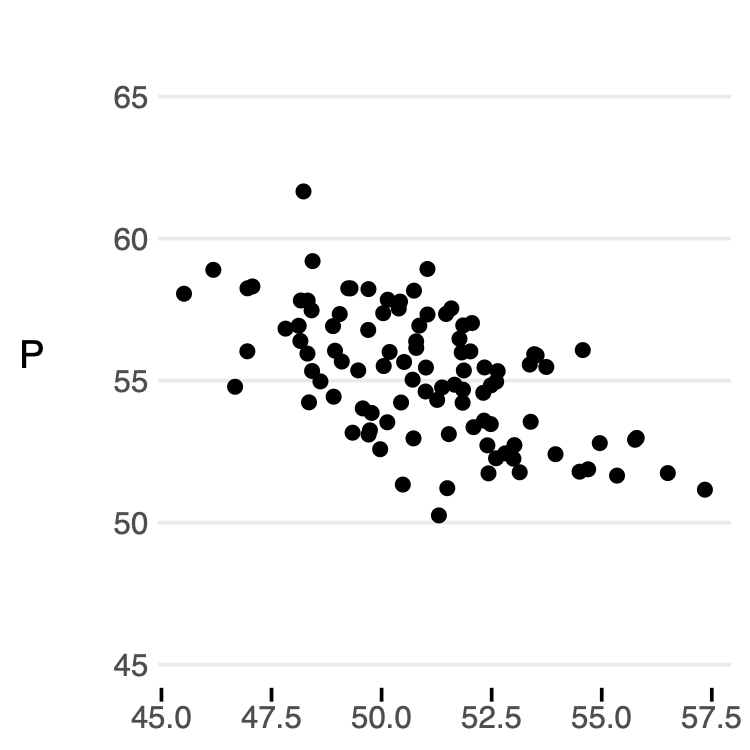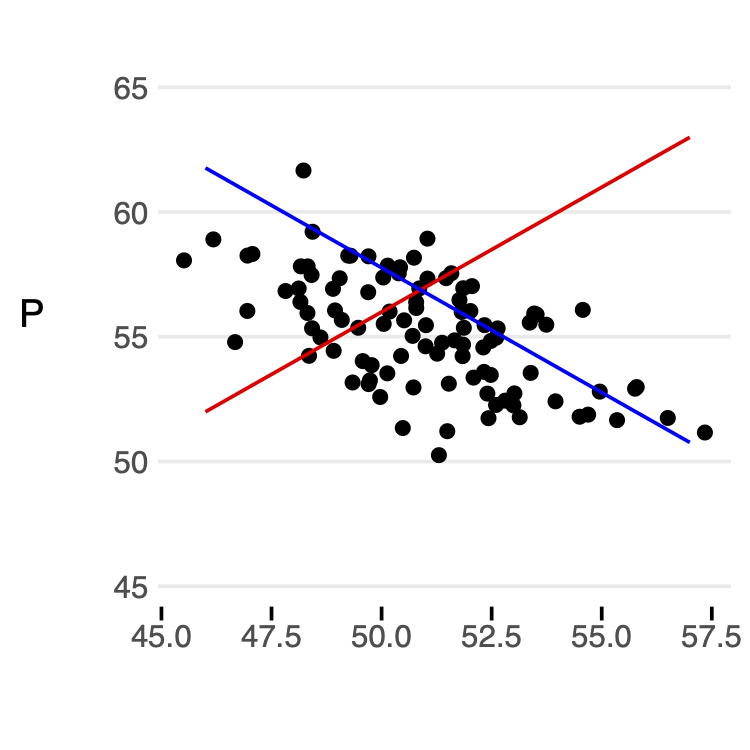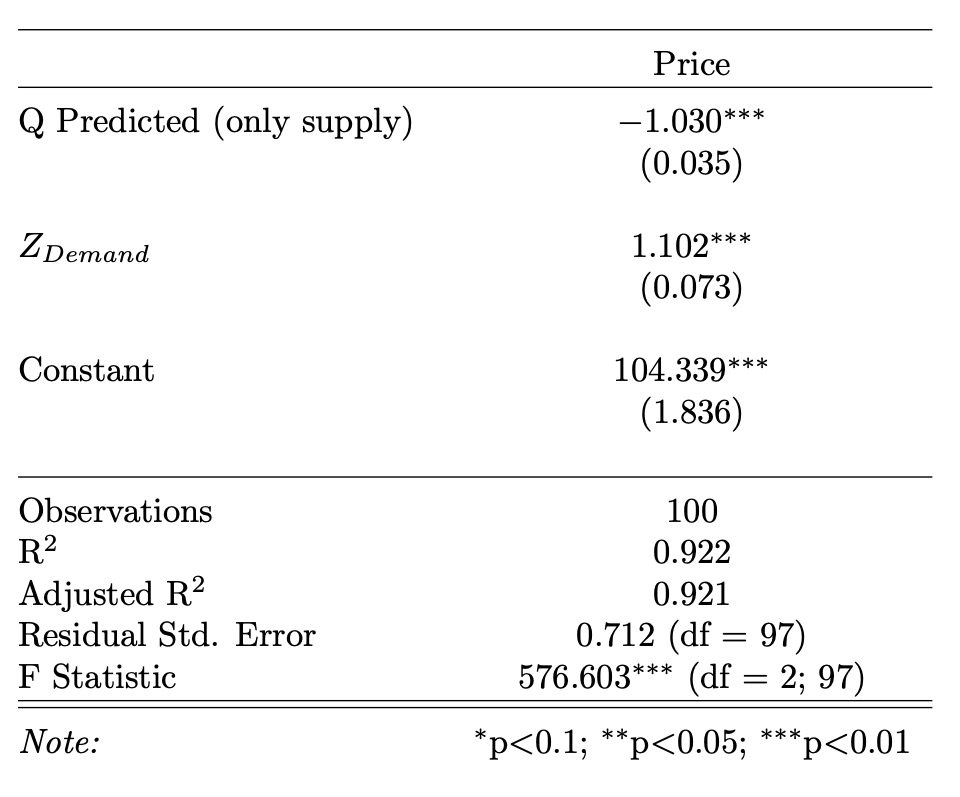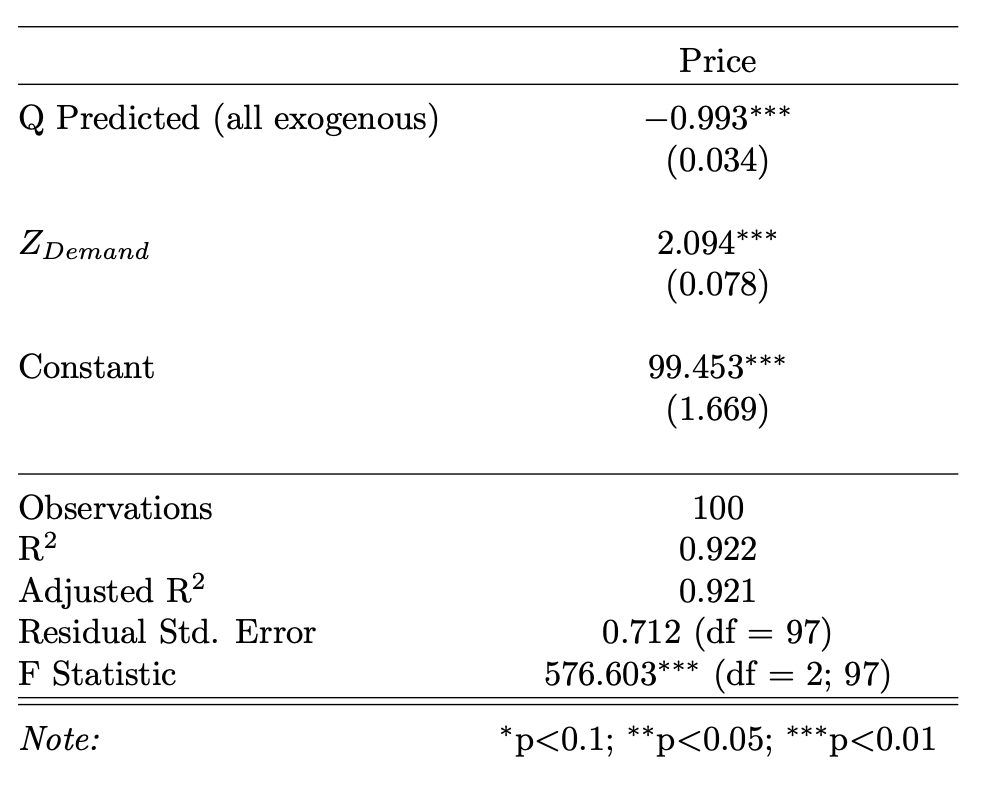## Overview

The goal is to:

1. Determine the correct setup for the first stage regression, and
2. Provide intuition for why that is the case.

## Microeconomic Theory

Suppose the true supply function for some product is given by price, and one (or more) exogenous supply shifers $$Z_{supply}$$. To make this concrete, consider the beef and steak products, where exogenous supply shifters would be inputs like the price of land, cattle feed, and so on.

\begin{aligned} Q_{supply} &= f(P_{supply}, \, Z_{supply}) \end{aligned}

and similarly, demand is given by:

\begin{aligned} Q_{demand} &= f(P_{demand}, \, Z_{demand}) \end{aligned}

If we observe transaction data in a competitive market, we will assume that prices and quantities are in equilibrium, thus implying:

$P_{supply} = P_{demand} = P^*$

and

$Q_{supply} = Q_{demand} = Q^*$

Together, we can find the reduced form equation for the equilibrium values:

\begin{aligned} Q_{demand} &= Q_{supply} \\ f(P^*, \, Z_{demand}) &= f(P^*, \, Z_{supply}) \\ \\ P^* &= f(Z_{demand}, Z_{supply}) \end{aligned}

Similarly, we can solve equilibrium quantity as:

\begin{aligned} f(Q^*, \, Z_{demand}) &= f(Q^*, \, Z_{supply}) \\ \\ Q^* &= f(Z_{demand}, Z_{supply}) \end{aligned}

$$\textcolor{red}{NOTE}$$: In equilibrium, price and quantity only depend on the exogenous supply and demand shifters.

## Simulation

Using this theory as a guide, we can build a simulation dataset with the following linear functional forms:

### Setup

\begin{aligned} P_{supply} &= Q_{supply} + 2Z_{supply} + u_{supply} \end{aligned}

and similarly, demand is given by:

\begin{aligned} P_{demand} &= 100 - Q_{demand} + 2Z_{demand} + u_{demand} \end{aligned}

Which gives us the reduced form relationships:

\begin{aligned} P^* = 50 + Z_{supply} + Z_{demand} + v \end{aligned}

Where $$v = \frac{u_{demand} - u_{supply}}{2}$$ contains the errors for both supply and demand.

### Build Dataset

Using this relationship, we can create a simulation dataset as follows:

1. Generate random values for $$u_{demand}$$, $$u_{supply}$$, $$Z_{demand}$$, and $$Z_{supply}$$.
2. Use these to find equilibrium prices.
3. Back out equilibrium quantities from the supply or demand equation (and verify that they are in fact the same).
4. Finally, we only keep positive values for price and quantity since a firm would simply shut down if that were the case.
# number of observations to generate
N <- 100

# ud and us are exogenous ~ N(0, 1)
ud <- rnorm(N)
us <- rnorm(N)
v <- (ud - us) / 2

# supply and demand shifters are exogenous normal
# although I believe the distributional assumptions
# can be relaxed
z1 <- rnorm(N) * 2 + 2  # ~N(2, 2)
z2 <- rnorm(N) + 3      # ~N(3, 0)

# price in equilibrium using reduced form equation
p <- 50 + z1 + z2 + v

# verify quantities are the same in equilibrium!
qd <- 100 - p + 2*z2 + ud
qs <- p - 2*z1 + us
q  <- qd

if (sum(qd - qs) > 1e10) {
cat("ERROR: Supply and demand not in equilibrium")
}

# make dataset
# we only keep values where quantiy and price
# are greater than zero. Our simulated datset may
# contain negative values since non-zero conditions
# are economic assumptions.
data <- tibble(
"Q" = q,
"P" = p,
"Z_Supply" = z1,
"Z_Demand" = z2
) %>%
filter(
Q > 0 && P > 0
)


### Graph

After generating a dataset according to those rules, we can view market prices and quantities in a scatter plot:

ymin <- min(data$P) - 5 ymax <- max(data$P) + 5
data %>%
ggplot(aes(Q, P)) +
geom_point() +
ylim(ymin, ymax) +
theme_wsj()If we lock in some value of $$Z_{demand}$$ and $$Z_{supply}$$, we can easily see how supply and demand intersect.

z1_fixed1 <- data$Z_Supply z1_fixed2 <- data$Z_Supply
z1_fixed3 <- data$Z_Supply z2_fixed1 <- data$Z_Demand
z2_fixed2 <- data$Z_Demand z2_fixed3 <- data$Z_Demand

data$Qs <- 1:dim(data) data$Ps1 <- data$Qs + 2 * z1_fixed1 data$Ps2 <- data$Qs + 2 * z1_fixed2 data$Ps3 <- data$Qs + 2 * z1_fixed3 data$Qd <- data$Qs data$Pd1 <- 100 - data$Qd + 2 * z2_fixed1 data$Pd2 <- 100 - data$Qd + 2 * z2_fixed2 data$Pd3 <- 100 - data$Qd + 2 * z2_fixed3 xmin <- min(data$Q)
xmax <- max(data$Q) ymin <- min(data$P) - 5
ymax <- max(dataP) + 5 data %>% ggplot(aes(Q, P)) + geom_point() + geom_line(aes(Qs, Ps1), color = "red") + geom_line(aes(Qd, Pd1), color = "blue") + xlim(xmin, xmax) + ylim(ymin, ymax) + theme_wsj()### Intuition for First Stage If we are trying to estimate demand elasticity by 2SLS, the naive regression of $P = \beta_1 Q + \beta_2 Z_{demand}$ We will get a biased estimate for $$\beta_1$$. Intuitively, this is because price and quantity were determined in equilibrium. In other words, conditional on the exogenous supply and demand shifters ($$Z_{demand}$$ and $$Z_{supply}$$), there is no variation in price or quantity. Instead, we will rely on exogenous variation that only impacts quantity through supply, in this case the supply shifters $$Z_{supply}$$. We can think of this as using exogenous shifts in the $$\textcolor{red}{red}$$ line to help determine the slope of the $$\textcolor{blue}{blue}$$ line. data %>% ggplot(aes(Q, P)) + geom_point() + geom_line(aes(Qs, Ps1), color = "red") + geom_line(aes(Qs, Ps2), color = "red", linetype = "dashed") + geom_line(aes(Qs, Ps3), color = "red", linetype = "dashed") + geom_line(aes(Qd, Pd1), color = "blue") + xlim(xmin, xmax) + ylim(ymin, ymax) + theme_wsj()Finally, why is that that we include the demand shifters in the first equation? Intuitively, this helps to isolate which level of the curves we are looking at. For example, for a given supply curve, we still need to include demand factors to let us pinpoint where we expect the levels to intersect. In other words, we need to know the external factors that are affecting the $$\textcolor{red}{red}$$ and $$\textcolor{blue}{blue}$$ lines. As shown above, in equilibrium, those are the only factors for price and quantity. ### Regression Results Finally, we test these ideas directly to see if we can recover our original “true” demand parameters. Recall, we are looking to retrieve the “true” values from the simulation setup, \begin{aligned} P_{demand} &= 100 - Q_{demand} + 2Z_{demand} + u_{demand} \end{aligned} #### Only Supply Shifters in First Stage If we only include supply shifters in the first stage regression, i.e. $Q = \alpha_1 Z_{supply} + u$ And use the predicted values, $$\hat{Q}$$ in the second stage regression: $P = \beta_1 \hat{Q} + \beta_2 Z_{demand} + e$ Then we obtain baised and inconsistent estimates. We can see the result of this estimation below: # first stage first_stage_bias_fit <- lm(Q ~ Z_Supply, data = data) dataQ Predicted (only supply) <- predict(first_stage_bias_fit)

# second stage
fit_bias <- lm(P ~ Q Predicted (only supply) + Z_Demand, data = data)


We see here that these estimates are incorrect based on what we know to be true.#### All Exogenous Variables in First Stage

If we now include all exogenous variables in the first stage regression, i.e.

$Q = \alpha_1 Z_{supply} + \alpha_2 Z_{demand} + u$

And use these new predicted values, $$\widetilde{Q}$$ in the second stage regression:

$P = \beta_1 \widetilde{Q} + \beta_2 Z_{demand} + e$

We can retrieve the known true paramater values.

# first stage
first_stage_fit <- lm(Q ~ Z_Supply + Z_Demand, data = data)
data\$Q Predicted (all exogenous) <- predict(first_stage_fit)

# second stage
fit <- lm(P ~ Q Predicted (all exogenous) + Z_Demand, data = data)In this case, using all exogenous variables in the first stage allows us to recover the “true” demand parameters on quantity and the demand shifter.